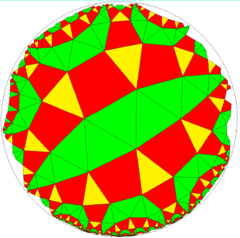# Snub order-6 square tiling

Snub tetratritetragonal tiling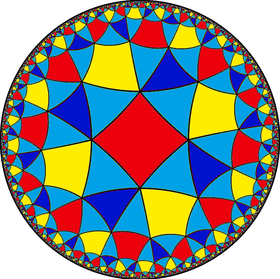Poincaré disk model of the hyperbolic plane
TypeHyperbolic uniform tiling
Vertex configuration3.3.3.4.3.4
Schläfli symbols(4,4,3)
s{4,6}
Wythoff symbol| 4 4 3
Coxeter diagram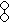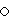Symmetry group[(4,4,3)]+, (443)
[6,4+], (4*3)
DualOrder-4-4-3 snub dual tiling
PropertiesVertex-transitive

In geometry, the snub tetratritetragonal tiling or snub order-6 square tiling is a uniform tiling of the hyperbolic plane. It has Schläfli symbol of s{(4,4,3)} or s{4,6}.

## Images

Drawn in chiral pairs: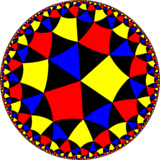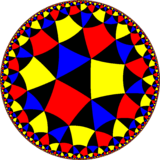## Symmetry

The symmetry is doubled as a snub order-6 square tiling, with only one color of square. It has Schläfli symbol of s{4,6}.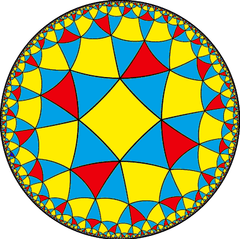## Related polyhedra and tiling

The vertex figure 3.3.3.4.3.4 does not uniquely generate a uniform hyperbolic tiling. Another with quadrilateral fundamental domain (3 2 2 2) and 2*32 symmetry is generated by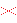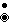: